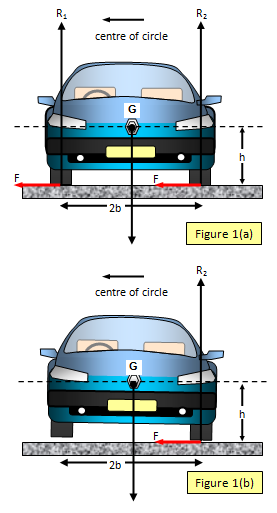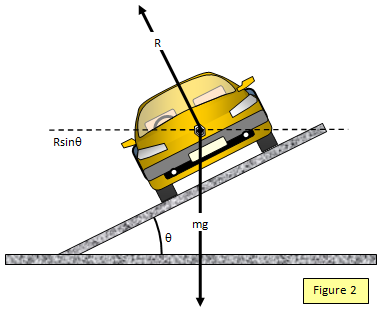## Car on flat track (unpowered)If a car corners on a flat road then the frictional forces between the car tyres and the road provide the centripetal force to make it take the curve. It is important to understand that there is not an additional force (mv2/r) - the frictional force is equal to the centripetal force - in actual fact it is the centripetal force.

The design of the car determines the maximum speed at which it can travel round a certain curve without tipping over. As you would expect the car with the lowest centre of gravity and the widest track will be able to corner the quickest. This is shown in the proof below where this maximum speed is found.

Let the height of the centre of gravity above the ground be h, the track width 2b, the speed of the car v, the radius of the curve r, and the mass of the car m. The reactions of the road of the inner and outer pairs of wheels are R1 and R2 respectively and the frictional force between the tyres and the road is F.
(See Figure 1(a)).

When the car is just about to fail to take the curve the inner wheels lift off the ground and so R1 will become zero. (See Figure 1(b)). The car will then tend to topple over outwards away from the centre of the corner.

Resolving vertically: R2 = mg
Taking moments about the centre of gravity :

R2b = Fh and so F = R2b/h = mgb/h

But since F = mv2/r we have mv2/r = mgb/h
and so the maximum speed at which the car can take the curve is given by :

Maximum speed (v) = [rgb/h]1/2

Notice that in the proof the car is considered to be unpowered. If the car is powered then an additional force is being applied in the direction of motion of the car at any instant and this will affect the maximum cornering speed.

Example problems
1. Calculate the minimum radius bend that an unpowered car travelling at 25 ms-1 can take without starting to topple over if the track width (2b) is 1.8 m, the height of its centre of gravity above the ground is 0.62 m.
Using: v2 = rgb/h and g = 9.8 ms-2
radius of curve (r) = v2h/gb = 252x0.62/9.8x0.9 = 43.9 m

2. Calculate the maximum speed at which a car can take a 25m radius curve without starting to topple over. (Track width = 1.8m, height of centre of gravity above the ground = 0.62m)

Using: v2 = rgb/h and g = 9.8 ms-2 maximum speed (v) =[25x9.8x0.9/0.62]1/2 = 18.9 ms-1 (41.5 mph)## Car on banked track (unpowered)

In this case it is the component of the weight of the car towards the centre of the curve that provides the centripetal force. Notice that there is no additional force mv2/r - the component of weight is the centripetal force. (See Figure 2)
If the track is banked at and angle q to the horizontal then:

Resolving horizontally and vertically:

Rsinq = mv2/r and Rcosq = mg

where v is the maximum speed that the car can take the corner.

so :
tanq = v2/rg

Notice that it can take the curve even if there is no friction between the tyres and the road.

Example problems
1. Calculate the maximum speed at which a car can travel on a frictionless banked track of radius 75 m if the angle of banking with the horizontal is 25o. (g = 9.8 ms-2)
Using: tanq= v2/rg          v2 = rgtanθ = 75x9.8xtan25 = 343
Giving maximum speed (v) = 18.5 ms-1.

2. Calculate the angle of banking needed on a test track of radius 200m if the car is to travel round at 120 mph (54.54 ms-1) without coming off the track. (g = 9.8 ms-2)
Using: tanθ= v2/rg tanθ = 54.542/[200x9.8] = 1.518
Therefore: Angle of banking (q) = 56.6o

A VERSION IN WORD IS AVAILABLE ON THE SCHOOLPHYSICS USB